# If f is the functions whose graph is shown, let h(x) = f(f(x)) and g(x) = f(x2). Use the graph of f to estimate the value of each derivative.b. g' (2)2.1a. h' (2)y= f(x)01х

Question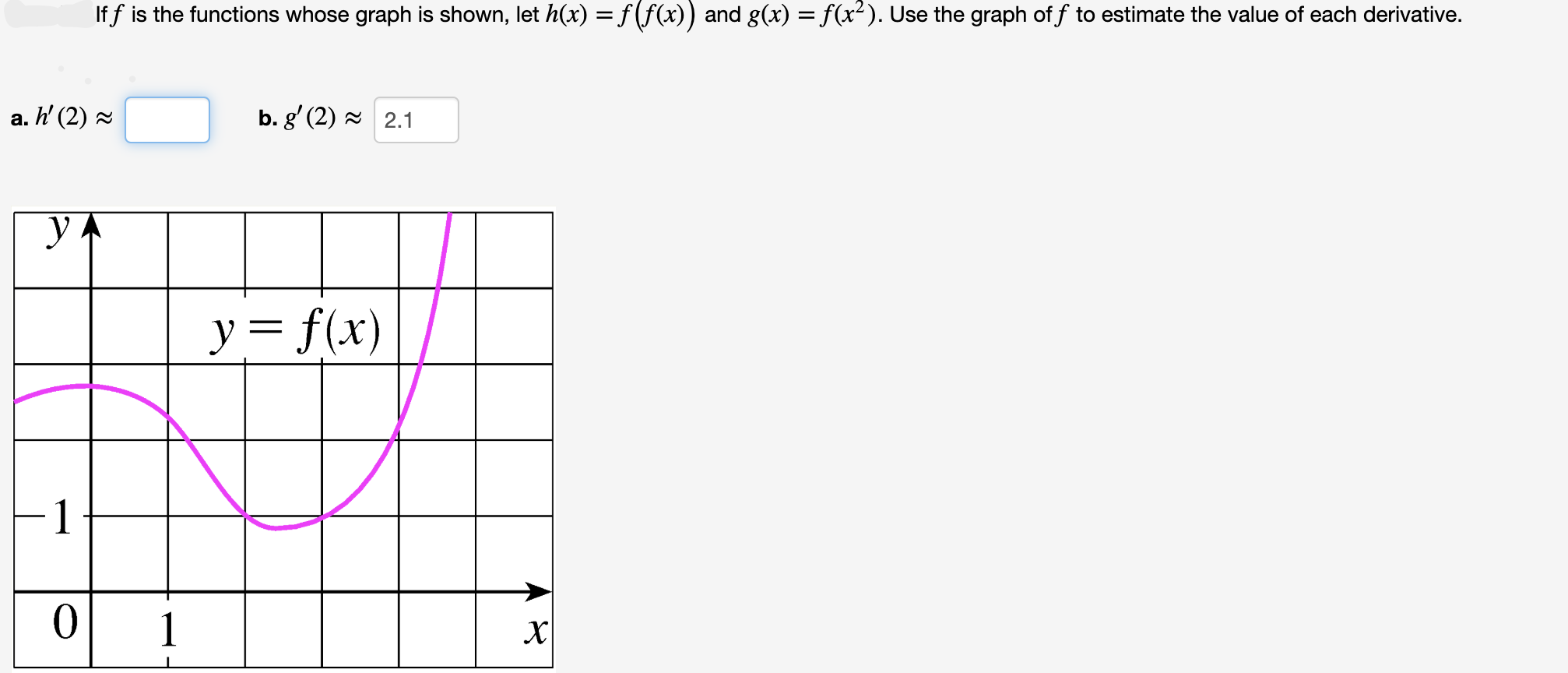help_outlineImage TranscriptioncloseIf f is the functions whose graph is shown, let h(x) = f(f(x)) and g(x) = f(x2). Use the graph of f to estimate the value of each derivative. b. g' (2)2.1 a. h' (2) y= f(x) 0 1 х fullscreen
check_circleExpert Solution
Step 1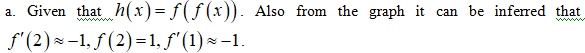Step 2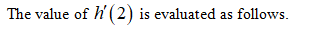Step 3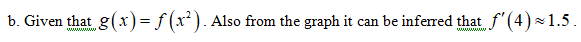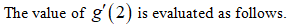...

### Want to see the full answer?

See Solution

#### Want to see this answer and more?

Solutions are written by subject experts who are available 24/7. Questions are typically answered within 1 hour*

See Solution
*Response times may vary by subject and question
Tagged in

### Derivative